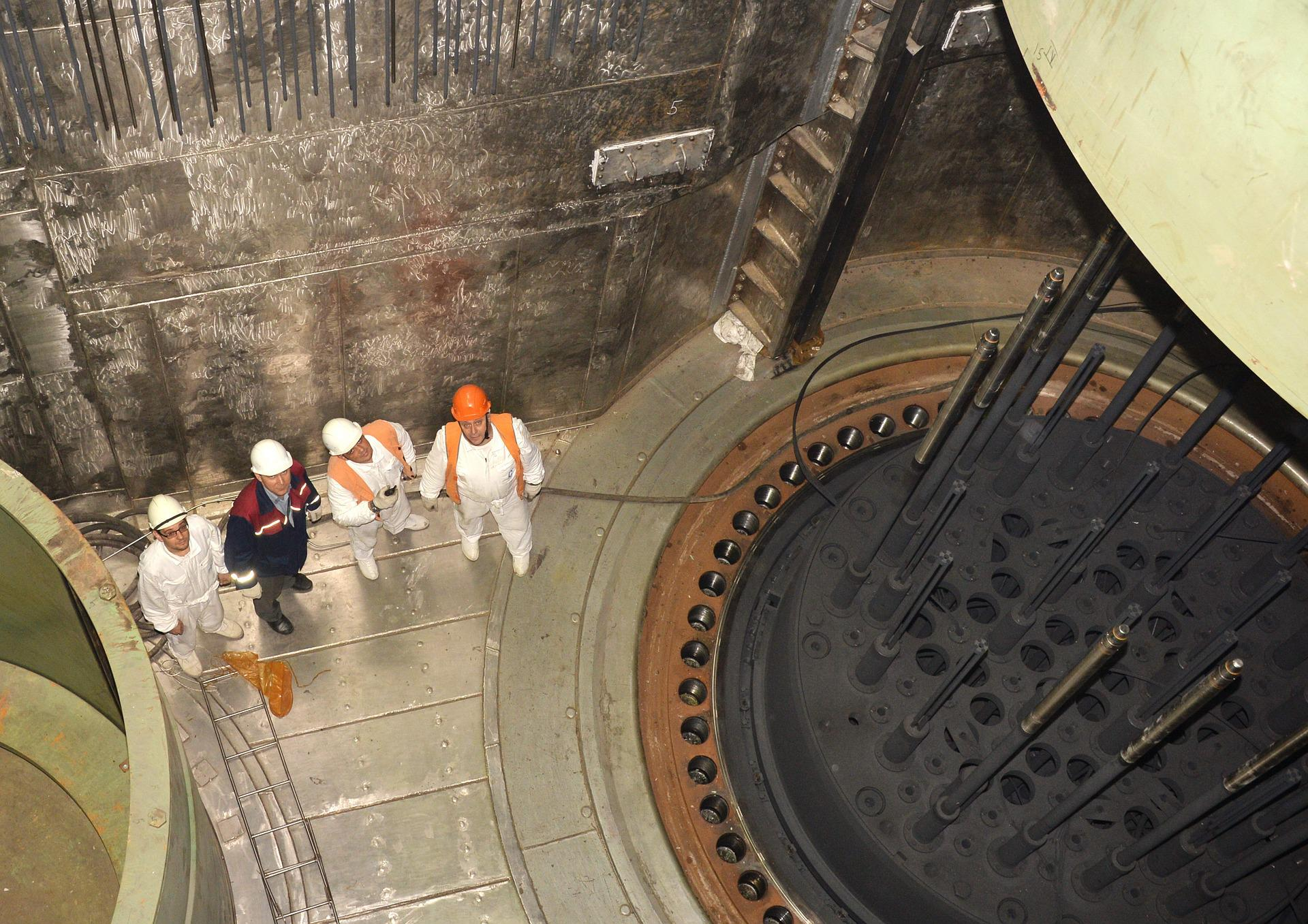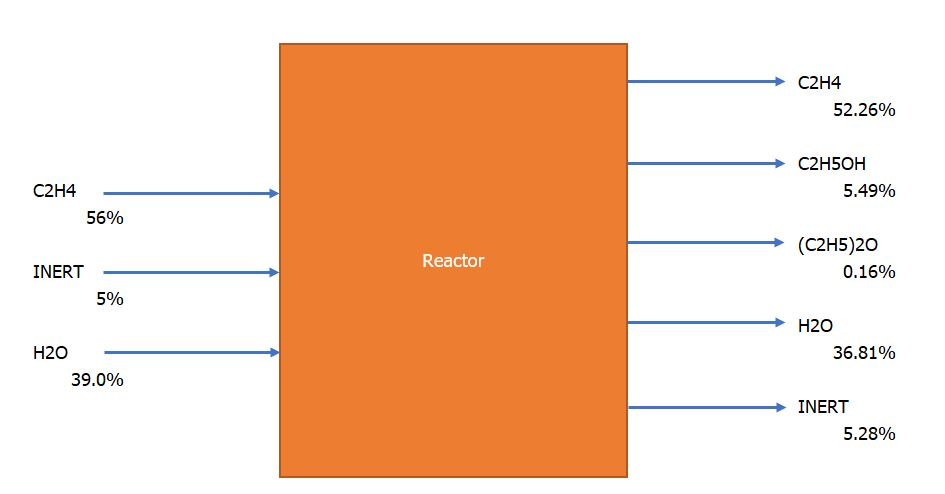# Difference between Conversion and Yield

It is important to differentiate between conversion and yield. Sometimes, these terms are mixed up. So, in this post, I want to share the difference between conversion and yield. I also will show you three examples of these terms.

Conversion is to do with reactants (reagents). On the other hand, yield is to do with products.

## Conversion

Conversion is a measure of the fraction of the reagent (reactant) that reacts. The conversion of a particular reagent is often less than 100% to optimize reactor design and to minimize formation of by-product.

If more than one reactant is used, the reagent on which the conversion is based must be specified.

Conversion is defined by the following equation:

This definition gives the total conversion of specific reagent to all products. Sometimes figures given for conversion refer to one specific product, usually the desired product. In this instance, the product must be specified as well as reagent/reactant. This is a really a way of expressing yield.

## Yield

Yield is a measure of the performance of a reactor or plant. Several different definitions of yield are used, and it is important to clearly state the basis of any yield figures. This is often not done when yield figures are quoted in the literature, and the judgement must be used to decide what was intended.

For a reactor, the yield is defined by:

There is term “reaction yield” (chemical yield), which includes only chemical losses to side product. And there is also “overall reactor yield” which includes physical losses.

Plant yield is a measure of the overall performance of the plant and includes all chemical and physical losses. Plant yield is expressed by this formula:

When more than one reagent is used, or product produced, it is essential that product and reagent to which the yield figure refers is clearly stated.

### Example 1 (Related to Conversion)

In the manufacture of vinyl chloride (VC, C2H3Cl) by pyrolysis of dichloroethane (DCE, C2H4Cl2), the reactor conversion is limited to 50% to reduce carbon formation. Calculate the quantity of DCE needed to produce 5000 kg/h VC.

Solution

Reaction:

C2H4Cl2 → C2H3Cl + HCl

Mol of C2H3Cl = mass flow rate/molecular weight = 5000/62.5 = 80 kmol/h

From the stoichiometric equation, 1 kmol C2H4Cl2 produces 1 kmol C2H3Cl. Therefore, required quantity of C2H4Cl2 = 80 / 50% = 160 kmol/h

### Example 2 (Related to Yield and Conversion)

In the production of ethanol by hydrolysis of ethylene, diethyl ether is produced as a by-product. A typical feed stream composition is: 56% ethylene, 5% inert, 40% water; and product stream: 52.26% ethylene, 5.49% ethanol, 0.16% ether, 36.81% water, and 5.28% inert. Calculate the yield of ethanol and ether based on ethylene.Reactions:

C2H4 + H2O → C2H5OH

2C2H5OH → (C2H5)2O + H2O

Basis: 100 mols feed.

As the inert will not react or converted, the amount will be the same at input and output.

Feed stream:

• C2H4 = 56 mol
• Inert = 5 mol
• H2O = 39 mol

Product:

• Inert = 5 mol (the same as inlet)
• C2H4 = (52.26% / 5.28%) × 5 mol = 49 mol
• C2H5OH = (5.49% / 5.28%) × 5 mol = 20 mol
• (C2H5)2O = (0.16% / 5.28%) × 5 mol = 15 mol
• H2O = (36.81% / 5.28%) × 5 mol =9 mol

Amount of C2H4 reacted or converted = amount of C2H4 in feed stream – amount of C2H4 in product stream = 56 – 49.49 = 6.51 mol

Yield calculation of C2H5OH based on C2H4:

• Yield of C2H5OH based on C2H4 = mols of C2H5OH produced × stoichiometric factor/mols of C2H4 reacted or converted
• Stoichiometric factor: 1 mol C2H4 required per mol C2H5OH produced
• Therefore, yield of C2H5OH based on C2H4 = 5.20 × 1 / 6.51 = 8%

Yield calculation of (C2H5)2O based on C2H4:

• Yield of (C2H5)2O based on C2H4 = mols of (C2H5)2O produced × stoichiometric factor/mols of C2H4 reacted or converted
• Stoichiometric factor: 1 mol C2H4 required per mol (C2H5)2O produced
• Therefore, yield of (C2H5)2O based on C2H4 = 0.15 × 2 / 6.51 = 65%

Conversion of C2H4 to all products = (amount of C2H4 in feed – amount of C2H4 in product) / amount of C2H4 in feed = (56 – 49.49) / 56 = 11.63%

### Example 3 (Related to Overall Yield and Chemical Yield)

In the chlorination of ethylene to produce dichloroethane (DCE), the conversion of ethylene is reported as 99%. If 93 mol of DCE are produced per 100 mol of ethylene fed, calculate the overall yield and the reactor (reaction) yield based on ethylene. The unreacted ethylene is not recovered.

Solution

Reaction:

C2H4 + Cl2 → C2H4Cl2

Stoichiometric factor is 1 (1 mol of C2H4 required for 1 mol of C2H4Cl2 produced).

There is difference between overall yield and chemical yield.

• Overall yield (including physical losses) = mol C2H4Cl2 produced × stoichiometric factor / mol C2H4 fed = 94 x 1 / 100 = 94%
• Chemical yield (reaction yield) = mol C2H4Cl2 produced × stoichiometric factor / mol C2H4 converted = 94 x 1 / 99 = 94.95%

I hope this quite long post is useful for you 😊